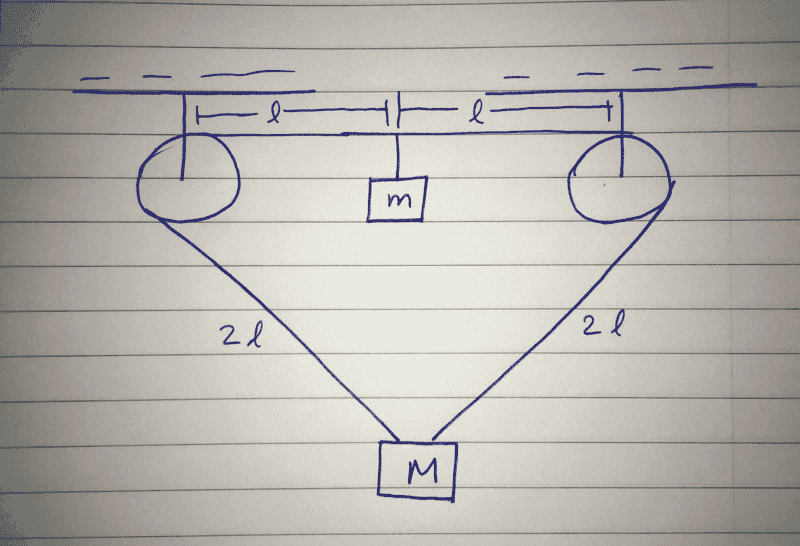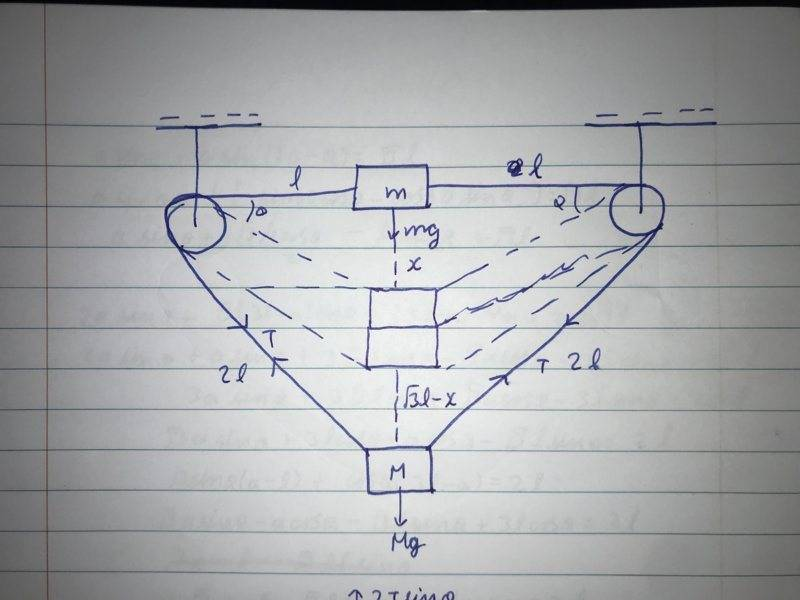# Energy: What will be the minimum ratio of m/M here

## Homework Statement

The objects are set free from the scenario shown in the attached image. Their masses have been given and they are under the effect of gravity. The length of the string has been shown in the figure. If the two blocks cross each other, then we need to determine the minimum value of m/M.

## Homework Equations

I am having a hard time creating equations. I am not able to determine how the equations can be formed for this question.

## The Attempt at a Solution

I tried using constraint, however, that was not of much help. I drew the diagram at intermediate and jotted down some equations with trigonometric ratios in them, but I wasn't able to make any conclusion.#### Attachments

•Delta2

berkeman
Mentor
Try drawing the two FBDs as a starting point...

I did, we will have 2Tsinθ on the object with mass M in the upward direction, I then drew an intermediate diagram too, but it is not working out.

berkeman
Mentor
I did, we will have 2Tsinθ on the object with mass M in the upward direction, I then drew an intermediate diagram too, but it is not working out.
Can you please Upload a copy of your FBDs (in PDF or JPEG format), and write out the equations that you have written for the problem? That will help us to help you. Thanks.This is the constraint diagram that I drew, this resulted in me writing a trigonometric equation that resulted in an error. I think I am making a mistake at the part where we are required to determine m/M such that they both "cross" each other, but I don't know for sure.#### Attachments

Homework Helper
Gold Member
If I understand it correctly, and I think I do, I think it is kind of simple. When they cross, the lengths will be equal on all of the lengths. You can even compute this length, and it is ## \frac{3}{2} L ##, because you have ## L_{total}=6L ##. In this case, when the diagram is symmetric, what happens if ## m>M ##? I think that is all this requires. The tension is symmetric around the pulleys. And it is not necessary to compute the tension or even the distance ## \frac{3}{2}L ##. Am I missing something? ## \\ ## The other thing I'm thinking though is that it requires a potential energy assessment, but scratch that=let's assume they cross each other and remain crossed.

Last edited:
berkeman
Mentor
@PhysicsBoi1908 -- Are the radii of the pulleys specified as zero, or at least implied? Also, do they say more explicitly what is meant by "crossing" of the masses? When they just touch top-to-bottom (in which case we need the vertical dimensions of each mass), or when their COMs cross? These questions change the geometry of the drawing...

That was bothering me too, however, I don't think that we will have to consider the pulley to have any radius. As for the objects crossing, we need to determine the minimum ratio of m/M, that leads me to think that we just need to have the objects touching each other, because if either of the masses will continue moving, then its mass will have to be relatively higher, but again, I don't know if I am right or wrong.

Homework Helper
Gold Member
It might interest you that from energy considerations, the results give that ##\frac{m }{M} \geq 2 \sqrt{\frac{3}{5}}-1 \approx .55 ## if I computed it correctly, for ## m ## to ever be able to get even with ## M ##. It's difficult to assess the academic level that this problem is, and what exactly they are looking for. From a balance of forces, the answer is almost obvious, but there are a couple of ways to interpret it. ## \\ ## For the energy case, it would not remain balanced in that position=the heavier mass would fall back down. ## \\ ## The solution using energy considerations actually makes it a more interesting problem, but it does require the student to know that potential energy ## U=mgh ##.

Last edited:
•PhysicsBoi1908 and Delta2
Are we supposing that the final diagram will be symmetric because both of them will have the same velocity?

Homework Helper
Gold Member
For the balanced force case, the masses come to a stop if the ratio is the minimum=the answer for that case is ## \frac{m}{M}=1 ##. ## \\ ## For the energy consideration case, when ## \frac{m}{M} ## is at the minimum, they will both be slowing down and become stationary as they meet, so that the kinetic energy of each is zero. The only energy that needs to be computed there is the change in gravitational potential for each. The more I look at this problem, the more I think they might be looking for the student to consider the energy of the system. The statement of the problem just says "if the two blocks cross each other". It doesn't say that they need to remain crossed.

Last edited:
•Delta2
Yes! Even I arrived at m/M=1, but that isn't the answer, I just had an idea, I will try it out and report back in a few minutes.

•Any ideas on how we can relate x and y to l individually? The relation: x+y= 3½ is a relation having both x and y.

Homework Helper
Gold Member
You are starting to get the idea, but it stil needs some additional computing. What is the height of the two as they meet? I get that the mass ## m ## drops by a distance of ## \frac{\sqrt{5}}{2} L ##. (Compute the y distance when the hypotenuse is ## \frac{3}{2} L ## ).## \\ ## Then you also need to compute how much mass ## M ## rises, etc. That is actually straightforward. It starts out down below by ## \sqrt{3} L ##.

How did the mass m drop by √5/2? Moreover, if it did, then we can say that since x+y=√3l, y=√3l-√5/2

Homework Helper
Gold Member
See my post 6 above. Hypotenuse=(3/2) L.

Oh ok, I will try using it.

Homework Helper
Gold Member
I corrected post 15=it needs an ## L ## multiplied by ## \frac{\sqrt{5}}{2} ##, i.e. ## x=\frac{\sqrt{5}}{2} L ##. You almost have the result. Check your arithmetic/algebra. I get ## \frac{m}{M}=2 \sqrt{\frac{3}{5}}-1 ##.

Homework Helper
Gold Member
The total length of the string is ## 6 L=2L+2L+L+L ##. You have 4 equal lengths, two on the left side and two on the right, going to the pulleys.

Oh, ok, I will try asking my teacher if the answer is correct and report back. Thank you for your help.

•Homework Helper
Gold Member
Additional comment: The clue is actually in the title of the thread that I am just looking at now="Energy". Looks like we might have interpreted it correctly.•Delta2 and PhysicsBoi1908
Seems like it , I have sent our answer to my teacher, he will respond when he will be free, hopefully within a few hours

•Delta2
Homework Helper
Gold Member
If you try to form the differential equations of motion for this problem, they are gonna be non linear cause as you already pointed out they ll contain term of ##\sin\theta(t)## and ##\tan\theta(t)## or possible square roots, so the only easy way I see is by energy methods as already Charles pointed out.

Last edited:
•PhysicsBoi1908
Yes, I tried forming such equations, but it only made the question more complex, the energy method is much nicer.

•Delta2
My sir hasn't replied yet, however, my friend has pointed out a mistake, according to what we have calculated, the ratio m/M is nearly 0.5, this signifies that M is greater than m, however, if that was to be the case, then the object of mass M will not go up, or will it because of initial tension?

Delta2
Homework Helper
Gold Member
It will go up, simply because the body of mass m will go down (the only vertical force that is acting at start is its weight, there is no vertical component of tension initially) and by going down it will pull the strings towards its side*, so the body of mass M will go up. The whole point is whether the body of mass M will go up enough so that they meet.

*Unless of course the strings can be stretched or broken but I am sure we are not examining this case here.

•